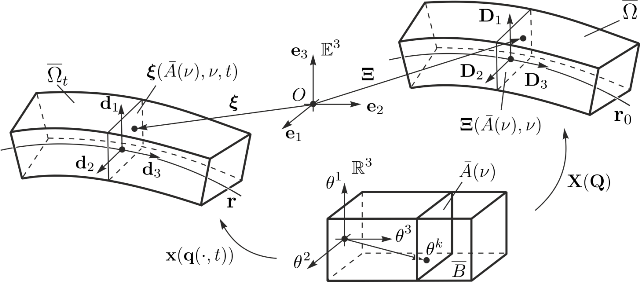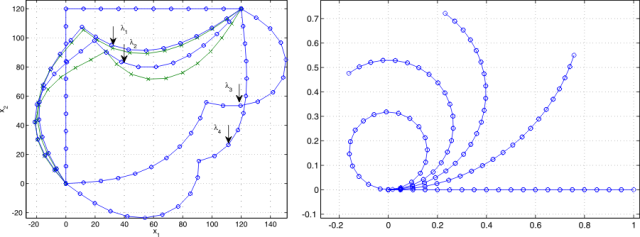# Induced beam theories and director-based beam finite elements

Even though computational performance is increasing, beam theories are still inevitable for many applications. The choice of a beam theory being the choice of a constrained position field reduces the space of possible deformation drastically.

By a variational formulation of the continuous body by its virtual work principle, the perfect constraint stress field, guaranteeing the restricted kinematics, is eliminated due to the principle of d'Alembert-Lagrange and the weak variational form of an appropriate beam theory is induced. Such an approach to beam theory relates the point of view of beams as generalized one-dimensional continua to the theory of continuous bodies. Using this procedure, all classical beam theories, where the cross sections remain rigid and plain, as well as augmented beam theories, where cross section deformation is allowed, can be derived.Reference and current configuration of a nonlinear Timoshenko beam

The weak variational form of an appropriate beam theory serves directly as the starting point of a finite element formulation. From a mechanical point of view, a finite element formulation is merely a further restriction of the beam kinematics such that the position field of the beam can be described by finitely many generalized coordinates. Due to the weak variational formulation, the constraint stress field of the discretization is eliminated. Since the beam theory is formulated for large displacements, also the finite element formulation is well suited for geometrical nonlinearities. In beam theories with rotational degrees of freedom a director based formulation with additional orthonormality constraints is proposed.Benchmark problems: Buckling test and bending problem

Publications

Eugster, S.R., Steigmann, D.J.: “Variational methods in the theory of beams and lattices”, in Encyclopedia of Continuum Mechanics, H. Altenbach, A. Öchsner (Eds.), Encyclopedia of Continuum Mechanics. Springer, pp. 1-9, 2018. PDF ECM

Eugster, S.R.: Geometric  Continuum Mechanics and Induced Beam Theories,Lecture Notes in Applied and Computational Mechanics Vol. 75, Springer-Verlag, 2015

Eugster, S.R.: “On the Foundations of Continuum Mechanics and its Application to Beam Theories“, PhD Thesis, ETH Zurich, 2014. PDF

Eugster, S.R., Hesch, C., Betsch, P. and Glocker, Ch.: “Director-based beam finite elements relying on the geometrically exact beam theory formulated in skew coordinates”, International Journal for Numerical Methods in Engineering, Vol. 97(2), pp. 111–129, 2014. PDF

### ContactDr. sc. ETH